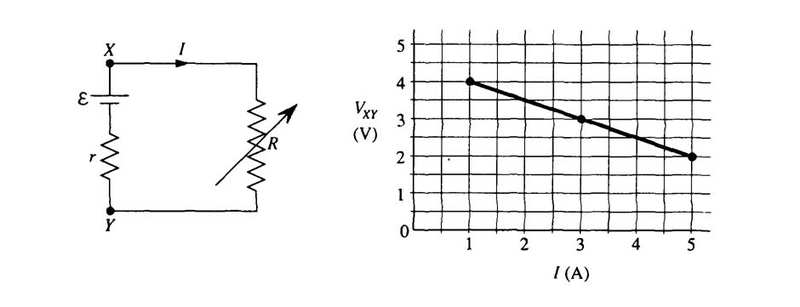# Electric Current

#### Kandycat

1. The problem statement, all variables and given/known dataA battery with emf, $$E$$, and internal resistance, r, is connected to a variable resistance, R, at points X and Y, as shown above on the left. Varying R changes, both the current I and the terminal voltage Vxy. The quantities I and Vxy are measured for several values of R and the data are plotted in a graph, as shown above the right.

(a) Determine the emf of the battery.

(b) Determine the internal resistance of the battery.

(c) Determine the value of the Resistance that will produce a current I of 3 amperes.

(d) Determine the maximum current that the batter can produce.

2. Relevant equations

V=IR

3. The attempt at a solution

I attempted to use Kirchhoff's loop rule. I'm not sure if I should be using it or not.

(a) $$E$$ - Ir - R = 0
$$E$$ = Ir + R

(b) r = ($$E$$ - R)/I

(c) V = IR
R = V/I = 3V/3A = 1 ohms

(d) I have no idea what to do.

Help?

#### Redbelly98

Staff Emeritus
Homework Helper
3. The attempt at a solution

I attempted to use Kirchhoff's loop rule. I'm not sure if I should be using it or not.

(a) $$E$$ - Ir - R = 0
$$E$$ = Ir + R
Instead of "R", that term should be "I R", the voltage across resistor R.

### The Physics Forums Way

We Value Quality
• Topics based on mainstream science
• Proper English grammar and spelling
We Value Civility
• Positive and compassionate attitudes
• Patience while debating
We Value Productivity
• Disciplined to remain on-topic
• Recognition of own weaknesses
• Solo and co-op problem solving